Further evidence against a force-velocity trade-off in a dynamic lever system

## Citation

Cox, Suzanne (2020), Further evidence against a force-velocity trade-off in a dynamic lever system, Dryad, Dataset, https://doi.org/10.5061/dryad.zpc866t71

## Abstract

Levers impose a force-velocity trade-off. In static conditions, a larger moment arm increases a muscle’s force capacity while a smaller moment arm enhances velocity at the end of the lever. However, muscle force is influenced by contractile velocity and fiber length. Additionally, the rate at which the muscle contracts is influenced by the inertial properties of the mass it accelerates. We hypothesize that these dynamic effects constrain the functional output of a muscle-lever system. We predict that there is an optimal moment arm to maximize output velocity for any given muscle-lever configuration. Here we test this hypothesis by building and systematically modifying a simple lever system in OpenSim. The model consists of a mass on the end of a lever that pivots around a pin joint, driven by a Millard2012EquilibriumMusclewith a non-compliant tendon. The muscle’s moment arm was defined by the radius of a cylinder which it wraps around. We generated 3600 modifications of this model with different muscles of varying optimal fiber lengths, moment arms and starting normalized muscle lengths. For each model we simulated the motion that results from 100% muscle activation and extracted the maximum velocity of the driven mass. In contrast to the common notion of a tradeoff between force and velocity in a lever system, we found that there was, instead, an optimal moment arm which maximized both velocity and total impulse. Increasing output velocity always requires increasing output force.  From this we conclude that in a dynamic lever system where muscle activation is held constant, there is no tradeoff between force and velocity, but, instead, an optimal relationship.

## Methods

We built a computational model of a simple lever system using OpenSim V. 4.0, scripting in MATLAB (MathWorks; Natick, MA).  Our model was composed of an output lever (length 0.12 m, width and height 0.04 m) that pivoted around a pin joint, a stand to connect the pin-joint to the world and a muscle that originated on the stand, wrapped around a wrap cylinder and attached to the lever (figure 1). These values were chosen to approximate the tarsometatarsus of the guinea fowl since detailed experimental data was available.   The diameter of the wrapping surface defined the input lever or moment arm of the muscle acting at this joint and allowed the muscle to change length and rotate the lever without altering the line of action. The muscle implemented was a Millard2012EquilibriumMuscle muscle model using default length-tension and force-velocity  values with a non-compliant tendon and a fixed pennation angle of zero.   The lever had the inertial properties of a rectangular rod defined by its volume with a density of water (997 kg m-3).  Gravity was not included in the model. To simulate the system driving a mass, we welded a mass on the end of the lever in the shape of a sphere with the density of water. We constrained the lever’s range of motion to 150 degrees with a joint limiting force stiffness of 20, damping of 5 and transition range of 2.5 degrees.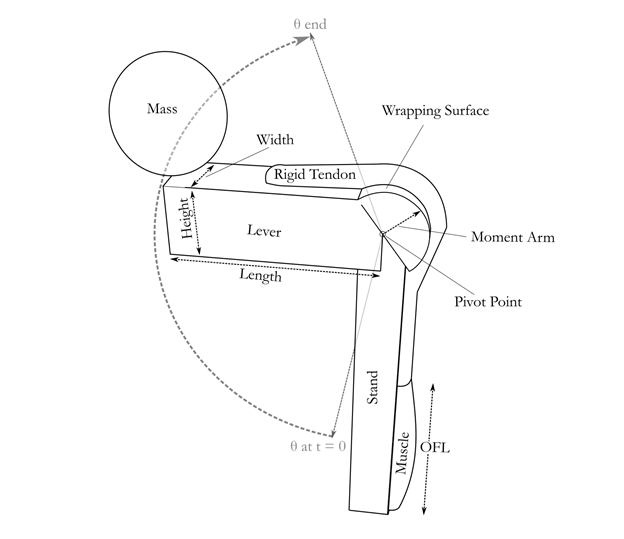We generated 3600 modifications of this model with different muscle optimal fiber lengths (OFL:(0.01:0.01:0.1 m), moment arms (0.0005:0.0005:0.02 m), starting normalized muscle lengths (0.8,1.1,1.4) and driven masses (0.01 ,0.5, 2 kg). Since muscle-tendon unit length changed with moment arm, we adjusted the tendon slack length for each model such that the equilibrium normalized fiber length for the passive muscle was within 0.01 units of a fixed starting fiber length. We held the volume of the muscle constant across changes in optimal fiber length.  Since the maximum isometric force of a muscle is a function of its cross-sectional area, iso-volumetric muscles imply that the force capacity, Fmax, is inversely related to optimal fiber length, OFL, such that

FmaxOFL = M Tsρ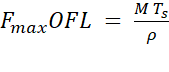,

where M is muscle mass, Ts is the specific tension of the muscle and ρ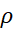is muscle density. The muscle was modeled on the sum of the lateral and medial gastrocnemius muscles of the guinea fowl, with a muscle mass of 17g, a specific tension of 3e5 N/m2 and a muscle density of 1060 kg/m3.  For each modified model, we simulated motion resulting from 100% activation of the muscle model across the full range of motion of the joint in 0.0005 second time steps.

At each time step we extracted the joint angle, angular velocity and acceleration of the lever as well as the muscle active-fiber force, fiber length, and fiber velocity. We trimmed this data to the time range over which the velocity of the mass was increasing. To distinguish the torque required to accelerate the lever from that available to accelerate the mass, we calculated them separately.  The force acting to accelerate the mass was calculated from the torque accelerating the mass, τm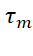, and the distance from the center of the mass to the pivot, d.

Fm=τmd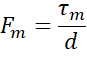The torque acting to accelerate the mass was calculated from the acceleration of the mass and its moment of inertia rotating around the pivot, calculated with the parallel axis theorem;

τm = 25 mr2+md2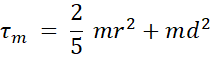where d is the distance from the center of mass to the pivot point,h r is the diameter of the added mass, and m is the mass of the added mass.  The torque acting to accelerate the lever was similarly calculated.

Additionally, impulse of the muscle and impulse applied to the mass were calculated as the integral of the active fiber force of the muscle and the force applied to the mass, respectively.

## Usage Notes

The code used to generate the data files

## Funding

Royal Society, Award: UF120507

UK MRC, Award: MR/T046619/1

Army Research Office, Award: W911NF-15-038